# Gravitation ppt.pdf

10 Feb 2023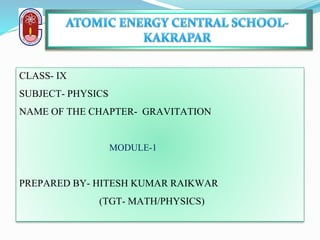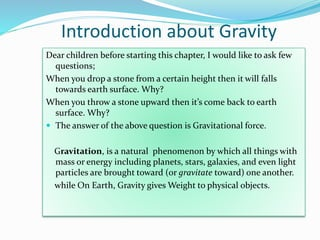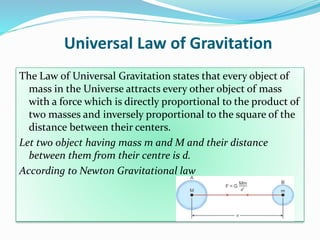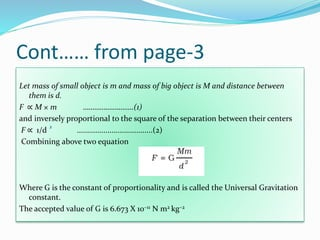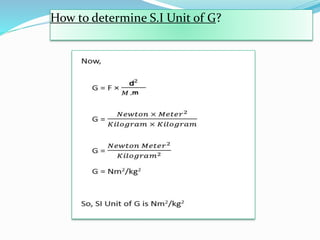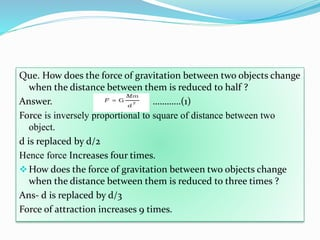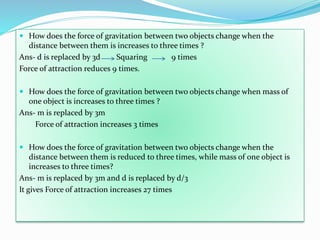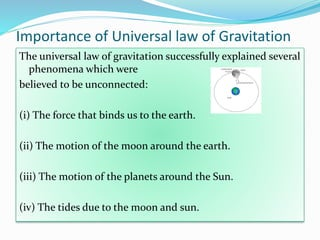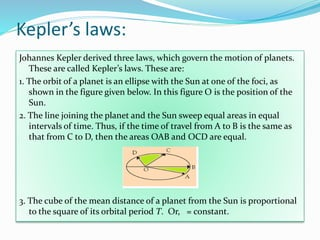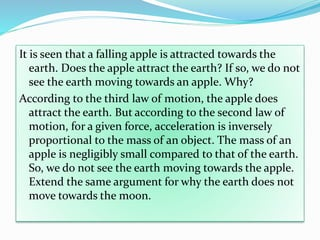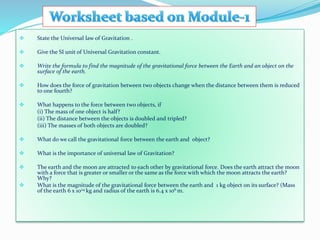1 sur 12

### Gravitation ppt.pdf

• 1. CLASS- IX SUBJECT- PHYSICS NAME OF THE CHAPTER- GRAVITATION MODULE-1 PREPARED BY- HITESH KUMAR RAIKWAR (TGT- MATH/PHYSICS)
• 2. Introduction about Gravity Dear children before starting this chapter, I would like to ask few questions; When you drop a stone from a certain height then it will falls towards earth surface. Why? When you throw a stone upward then it’s come back to earth surface. Why?  The answer of the above question is Gravitational force. Gravitation, is a natural phenomenon by which all things with mass or energy including planets, stars, galaxies, and even light particles are brought toward (or gravitate toward) one another. while On Earth, Gravity gives Weight to physical objects.
• 3. Universal Law of Gravitation The Law of Universal Gravitation states that every object of mass in the Universe attracts every other object of mass with a force which is directly proportional to the product of two masses and inversely proportional to the square of the distance between their centers. Let two object having mass m and M and their distance between them from their centre is d. According to Newton Gravitational law
• 4. Cont…… from page-3 Let mass of small object is m and mass of big object is M and distance between them is d. F ∝ M × m ………………………(1) and inversely proportional to the square of the separation between their centers F ∝ 1/d .....................................(2) Combining above two equation Where G is the constant of proportionality and is called the Universal Gravitation constant. The accepted value of G is 6.673 X 10–11 N m2 kg–2 2
• 5. How to determine S.I Unit of G?
• 6. Que. How does the force of gravitation between two objects change when the distance between them is reduced to half ? Answer. …………(1) Force is inversely proportional to square of distance between two object. d is replaced by d/2 Hence force Increases four times. ❖How does the force of gravitation between two objects change when the distance between them is reduced to three times ? Ans- d is replaced by d/3 Force of attraction increases 9 times.
• 7.  How does the force of gravitation between two objects change when the distance between them is increases to three times ? Ans- d is replaced by 3d Squaring 9 times Force of attraction reduces 9 times.  How does the force of gravitation between two objects change when mass of one object is increases to three times ? Ans- m is replaced by 3m Force of attraction increases 3 times  How does the force of gravitation between two objects change when the distance between them is reduced to three times, while mass of one object is increases to three times? Ans- m is replaced by 3m and d is replaced by d/3 It gives Force of attraction increases 27 times
• 8. The universal law of gravitation successfully explained several phenomena which were believed to be unconnected: (i) The force that binds us to the earth. (ii) The motion of the moon around the earth. (iii) The motion of the planets around the Sun. (iv) The tides due to the moon and sun. Importance of Universal law of Gravitation
• 9. Kepler’s laws: Johannes Kepler derived three laws, which govern the motion of planets. These are called Kepler’s laws. These are: 1. The orbit of a planet is an ellipse with the Sun at one of the foci, as shown in the figure given below. In this figure O is the position of the Sun. 2. The line joining the planet and the Sun sweep equal areas in equal intervals of time. Thus, if the time of travel from A to B is the same as that from C to D, then the areas OAB and OCD are equal. 3. The cube of the mean distance of a planet from the Sun is proportional to the square of its orbital period T. Or, = constant.
• 10. It is seen that a falling apple is attracted towards the earth. Does the apple attract the earth? If so, we do not see the earth moving towards an apple. Why? According to the third law of motion, the apple does attract the earth. But according to the second law of motion, for a given force, acceleration is inversely proportional to the mass of an object. The mass of an apple is negligibly small compared to that of the earth. So, we do not see the earth moving towards the apple. Extend the same argument for why the earth does not move towards the moon.
• 11. ❖ State the Universal law of Gravitation . ❖ Give the SI unit of Universal Gravitation constant. ❖ Write the formula to find the magnitude of the gravitational force between the Earth and an object on the surface of the earth. ❖ How does the force of gravitation between two objects change when the distance between them is reduced to one fourth? ❖ What happens to the force between two objects, if (i) The mass of one object is half? (ii) The distance between the objects is doubled and tripled? (iii) The masses of both objects are doubled? ❖ What do we call the gravitational force between the earth and object? ❖ What is the importance of universal law of Gravitation? ❖ The earth and the moon are attracted to each other by gravitational force. Does the earth attract the moon with a force that is greater or smaller or the same as the force with which the moon attracts the earth? Why? ❖ What is the magnitude of the gravitational force between the earth and 1 kg object on its surface? (Mass of the earth 6 x 1024 kg and radius of the earth is 6.4 x 106 m.